# Ratios

Reduce the numbers:

50 in a 1:2 ratio
111 at a ratio of 2:3
70 at 10:50
560 at a ratio of 3:8

a =  25
b =  74
c =  14
d =  210

### Step-by-step explanation:Did you find an error or inaccuracy? Feel free to write us. Thank you!Tips to related online calculators
Check out our ratio calculator.

## Related math problems and questions:

• RemaindersIt is given a set of numbers { 170; 244; 299; 333; 351; 391; 423; 644 }. Divide this numbers by number 66 and determine set of remainders. As result write sum of this remainders.
• Divide moneyDivide 1200 USD at a ratio of 1:2:3:4:5:6:9:10
• A bottle 2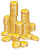A bottle contain 255 coins 1/3 of the coins are £1 cons 110 of the coins are 50p coins the rest of the coins are 20p coins. What is the total value of the coins contained in the bottle.
• DriverI drive at an average speed of 70km/h, I drive 10 thousand km. How long did it take me?
• Medicament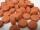The same type of medicament produces a number of manufacturers in a variety of packages with different content of active substance. Pack 1: includes 60 pills of 600 mg of active substance per pack cost 9 Eur. Pack 2: includes 150 pills of 500 mg of active
• RatiosDivide: a) 250 CZK in the ratio 2:3 b) 1000 CZK in the ratio 4:7:9
• Ratio6 numbers are in the ratio 1:5:1:5:5:5. Their sum is 242. What are the numbers?
• Kilo candyOne kilogram of chocolate candy cost 10 euros. Kate buys 250 grams and had the 8 pack of candy. How much does cost one bonbon?
• There 14There are 250 people in a museum. 2/5 of the 250 people are girls 3/10 of the 250 people are boys The rest of the 250 people are adults Work out the number of adults in the museum.
• Ratio - proportion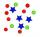Reduce the number 16 in proportion 3:2 5:4 11:8
• Washer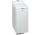The washing machine drum washes at 71 RPM. The washing machine motor pulley has a diameter of 8 cm. What must be the diameter of the drum machine pulley when the motor is at 351 RPM?
• BuildingAt the building, we divided 240 boards into two piles in a 5: 3 ratio. How many were fewer boards in the lower pile?
• Moneys in triple ratioMilan, John and Lili have a total 344 euros. Their amounts are in the ratio 1:2:5. Determine how much each of them has?
• The sidewalk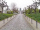The sidewalk is 50 m long. What will be its length on the plot plan in the scale of 1: 200?
• Three workersThree workers got paid for work. First earn € 50, the second € 100 and third € 60. For further work should receive together € 735. How do you divide that amount in order to be distributed in the same proportion as the first job?
• ConcertOn a Concert were sold 150 tickets for CZK 360, 235 tickets for 240 CZK and 412 for 180 CZK. How much was the total revenues for tickets?
• Electric energyThere are 875 identical light bulbs in the sports hall lighting for 2 hours. How long does the same amount of electricity consume 100 such bulbs?